# Wordcount

Github地址 https://github.com/iron-man45/WordCount

一、结对讨论的照片二、结对的PSP表格。

 PSP2.1 Personal Software Process Stages 预估耗时（分钟） 实际耗时（分钟） Planning 计划 15 30 · Estimate · 估计这个任务需要多少时间 30 30 Development 开发 360 360 · Analysis · 需求分析 (包括学习新技术) 60 90 · Design Spec · 生成设计文档 45 45 · Design Review · 设计复审 (和同事审核设计文档) 60 60 · Coding Standard · 代码规范 (为目前的开发制定合适的规范) 60 90 · Design · 具体设计 60 60 · Coding · 具体编码 300 300 · Code Review · 代码复审 60 60 · Test · 测试（自我测试，修改代码，提交修改） 60 30 Reporting 报告 30 30 · Test Report · 测试报告 30 30 · Size Measurement · 计算工作量 30 45 · Postmortem & Process Improvement Plan · 事后总结, 并提出过程改进计划 30 45 合计 1230 1305

a)    （ 解题思路描述。即刚开始拿到题目后，如何思考，如何找资料的过程。）刚得到题目，分析它的难易程度时，发现查找到单词以及词组运用正则运算来匹配

b)     （设计实现过程。设计包括代码如何组织，比如会有几个类，几个函数，他们之间关系如何，关键函数是否需要画出流程图？单元测试是怎么设计的？）

c)     （给出你们制定的代码规范或链接，记录你们代码互审的情况，审查的模块名、发现的问题等。）

listdo函数是分割字符串，判断是否是分割符时，‘>=’写成了'>'，不过一看单词的数量不对就很快的找到了这个错误。

d)     （记录在改进程序性能上所花费的时间，描述你改进的思路，并展示一张性能分析图（由VS 2017的性能分析工具自动生成），并展示你程序中消耗最大的函数）

e)     （代码说明。展示出项目关键代码，并解释思路与注释说明。）

public static List<string> WordN(string[] ma,int h)
{

List<string> sd = new List<string>();

List<string> sdx = new List<string>();//建立一个存储词组的列表
int i = 0;
foreach (string s in ma)               //遍历集合中每个单词
{
if(s!==""){
if(s.Length<4)
{
}
else
{
int j = 1;
for (i = 0; i < 4; i++)
{
if (((s[i] >= 'a') && (s[i] <= 'z')) || ((s[i] >= 'A') && (s[i] <= 'Z')))
{
}
else
{
j = 0;
break;
}
}
if (j == 1)
{
string s2 = s.ToLower();

}
else
{
}

}}

}
sdx.Add("!no");
if (h == 0) return sd; else return sdx; }

public static List<string> WordsCount(List<string> sdx,int n)
{
int i = 0;
List<string> ac = new List<string>();

foreach (string s in sdx)
{
if(s=="!no")
{
while(i>=n)
{
string ae="";
int j = 0;
{
ae+=af+" ";
j++;
if(j ==n)
{
break;
}
}
i--;
}
i = 0;
}
else
{
i++;
}

}

return ac;
}


 public static void ReadTxt(string path,string outpath,int n,int number,int a,int b)
{

Dictionary<string, int> e = new Dictionary<string, int>();//筛选后的词典



if (outpath != "out")
{

StreamWriter sw = new StreamWriter(outpath, false);//true表示追加
sw.WriteLine("characters: " + a);
sw.WriteLine("words: " + WordN(WordNumber(restOfStream), 0).Count());
sw.WriteLine("lines: " + b);

//输出单词
if (number > 0)
{
e = Chosewords(Listdo(WordN(WordNumber(restOfStream), 0)), number);
foreach (string s in e.Keys)
{

sw.WriteLine("<" + s + ">: " + e[s]);
}
}
if (n > 0)
{

List<string> ac = WordsCount(WordN(WordNumber(restOfStream), 1), n);

for (int k=0;k<ac.Count();k++)
{
if(ac[k] != "!no")
{
string xs = ac[k];

sw.Write(xs);
int numa = 0;
for(int u=0;u<ac.Count();u++)
{
if (ac[u] == xs)
{
ac[u] = "!no";
numa++;
}
}

sw.WriteLine(": " + numa);
}
}

}

sw.Flush();
sw.Close();
}

}

static void Main(string[] args)
{
string p ="in";//输入文件路径
string q="out";//输出文件路径
int n=0;//词组长度
int number=0;//输出单词个数
for (int i=0;i<args.Length;i++)
{
switch (args[i])
{
case "-i":p = args[i + 1];break;
case "-o": q = args[i + 1]; break;
case "-m": n=int.Parse(args[i+1]); break;
case "-n": number= int.Parse(args[i+1]); break;
}
i++;
}

//计算字符数汉字除外
int a = FirstCount(p,0);
//计算非空行数
int b = FirstCount(p,1);
//输出到目标文件

}

f)       （结合在构建之法中学习到的相关内容与结对项目的实践经历，撰写解决项目的心路历程与收获，以及结对感受，是否1+1>2）两个人做项目的速度明显是比一个

创建单元测试，这里只展示出错的函数测试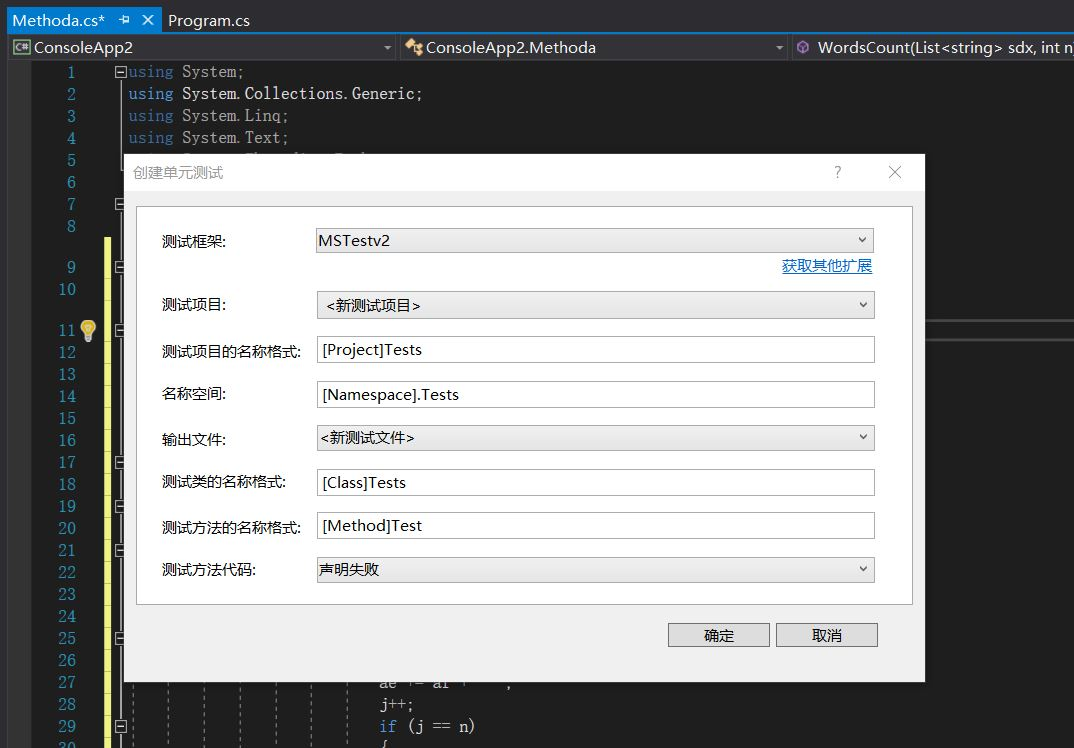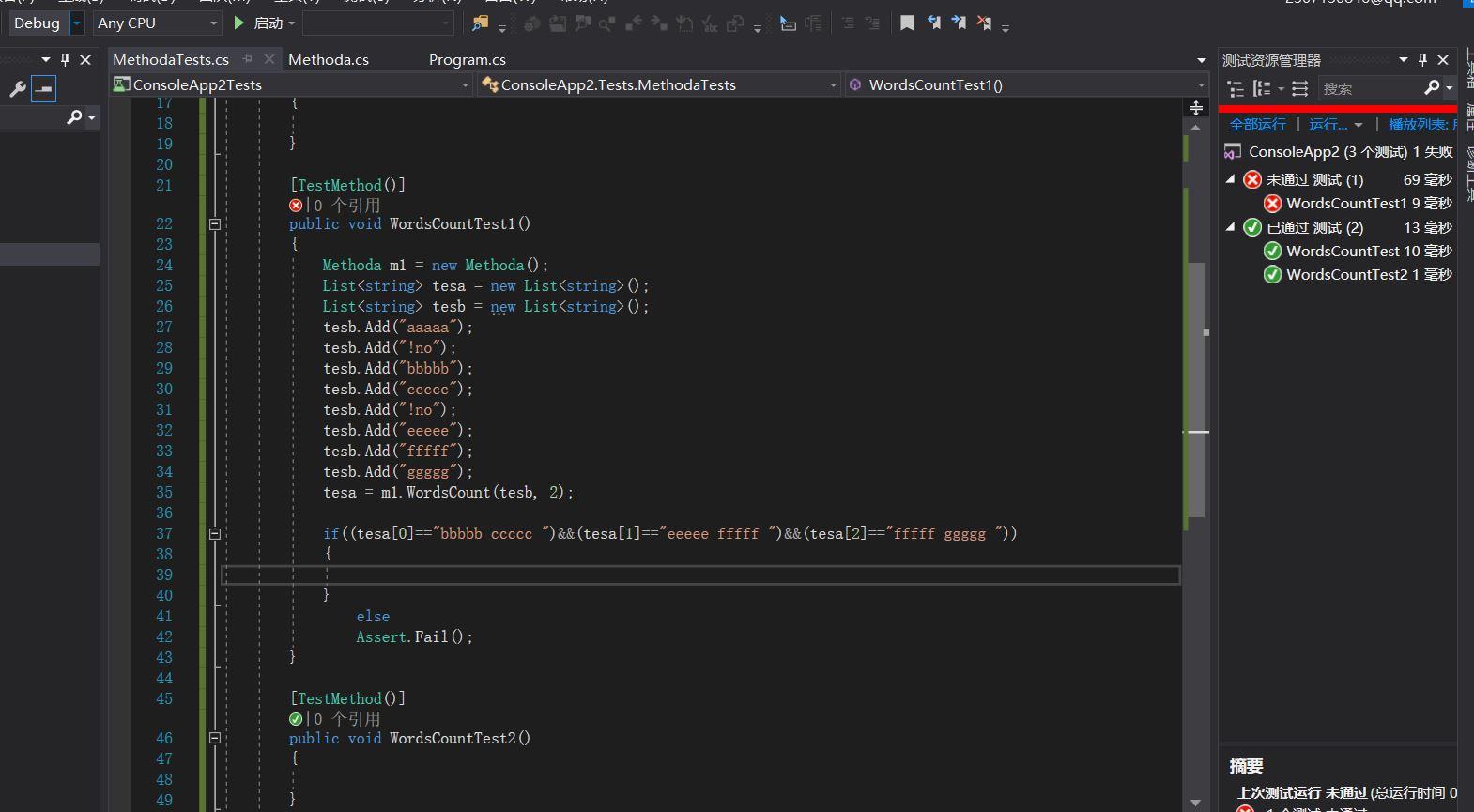第一个问题是读取文件时没有使用utf8编码，导致汉字占用了后面单词的空间，改变了单词的长度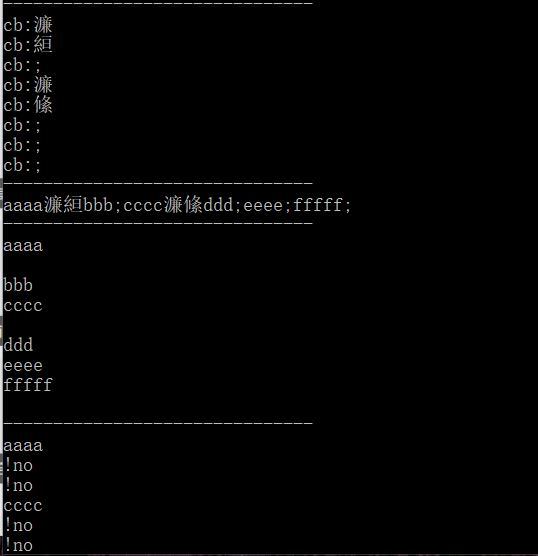不过及时地找出了这个问题的原因，并且解决了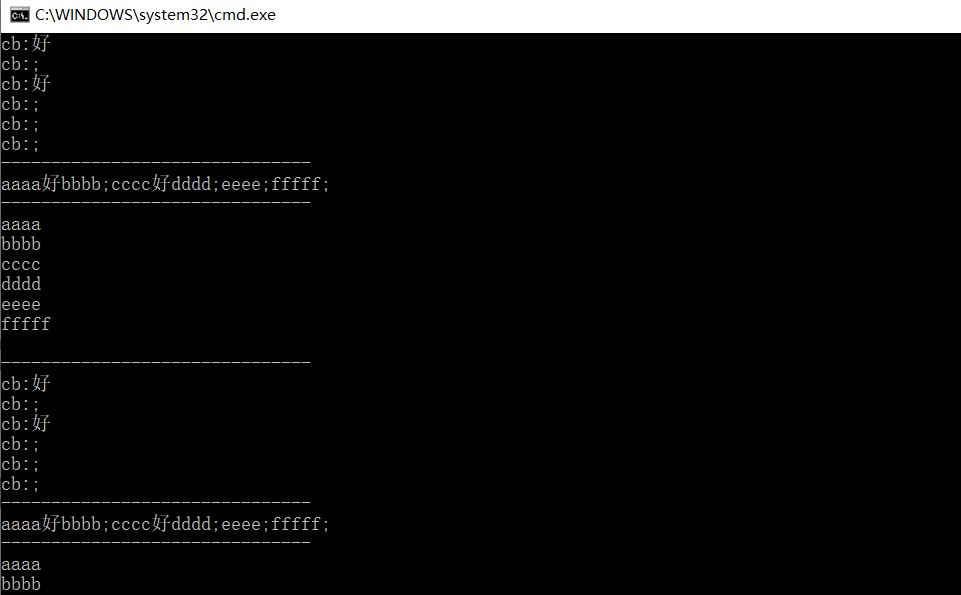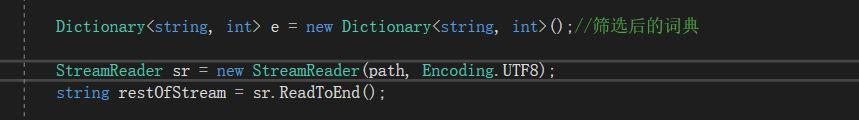然而解决完上面这个问题，新的问题又出现了，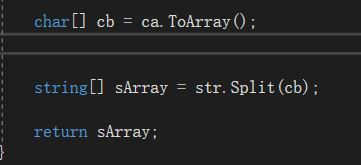我为了找出问题，把字符串数组换行输出，发现数组存在空字符串，这才解决了问题。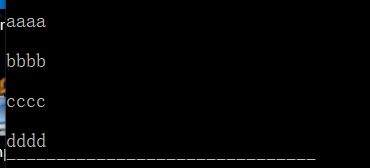最终测试通过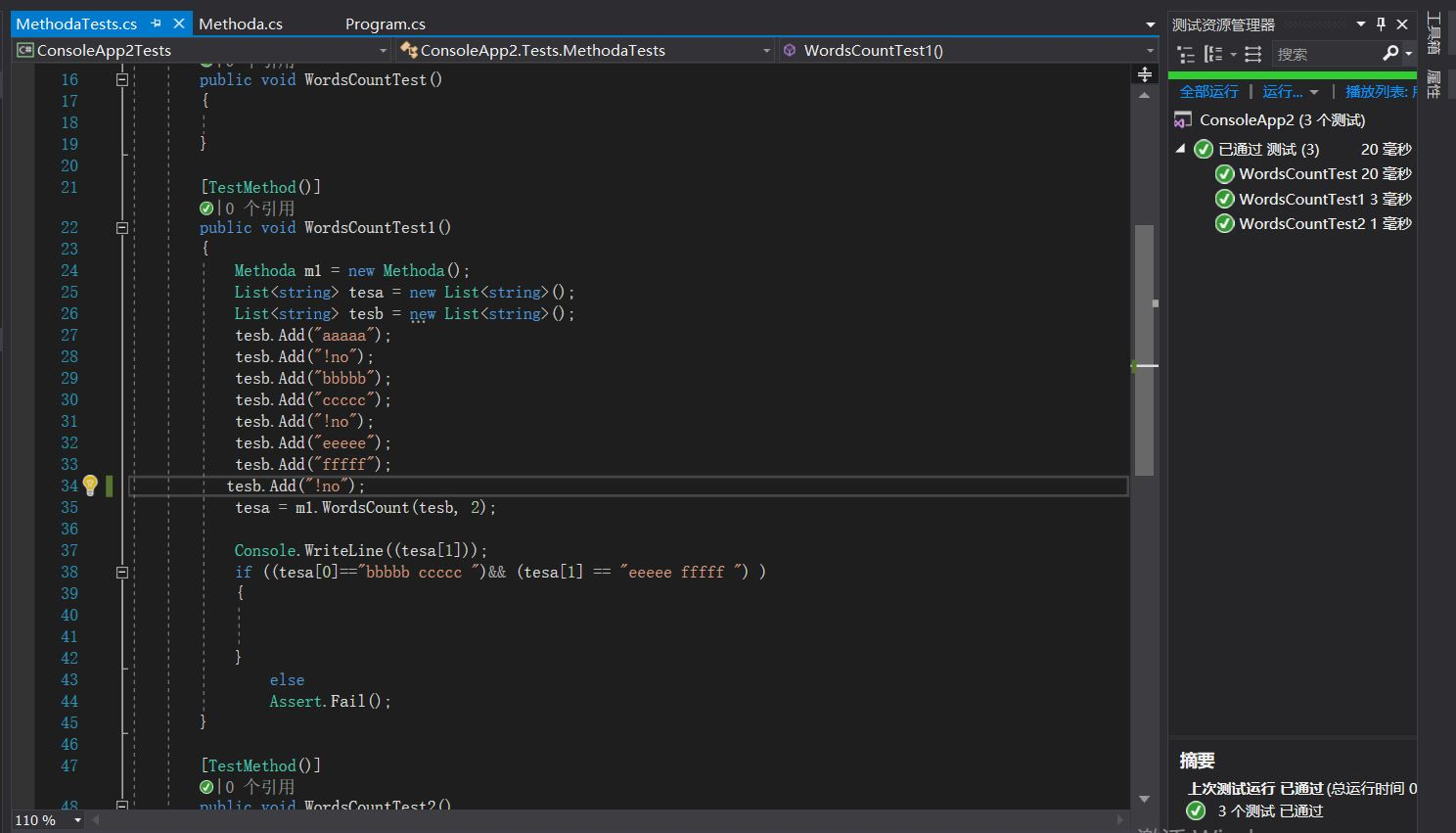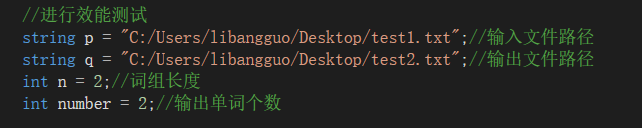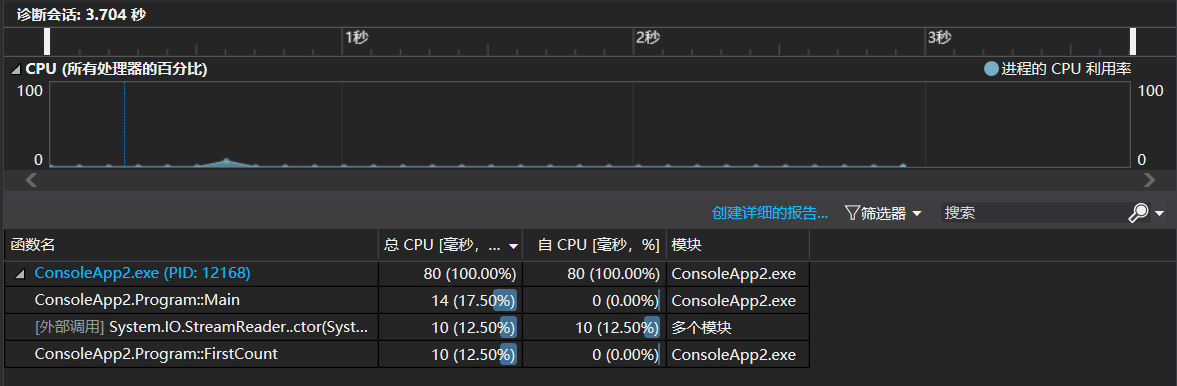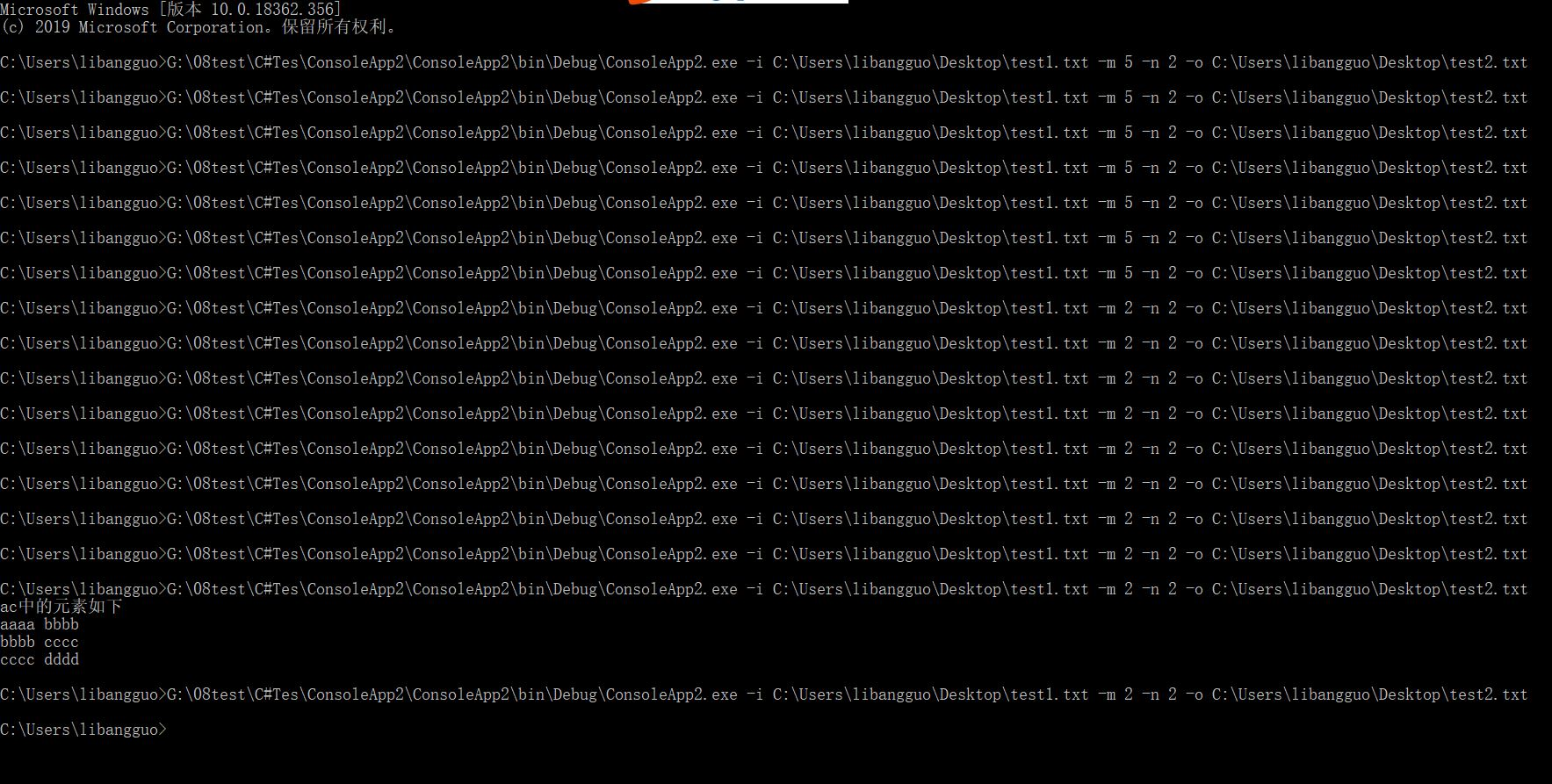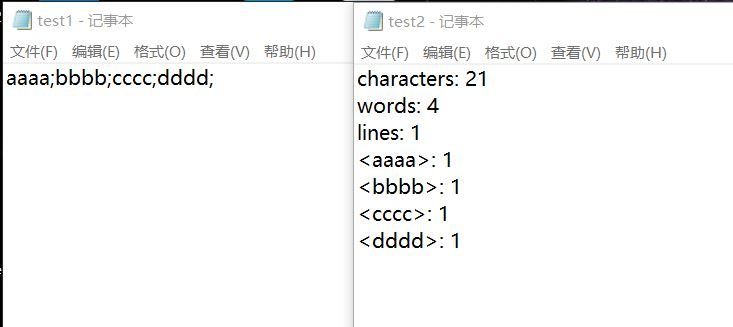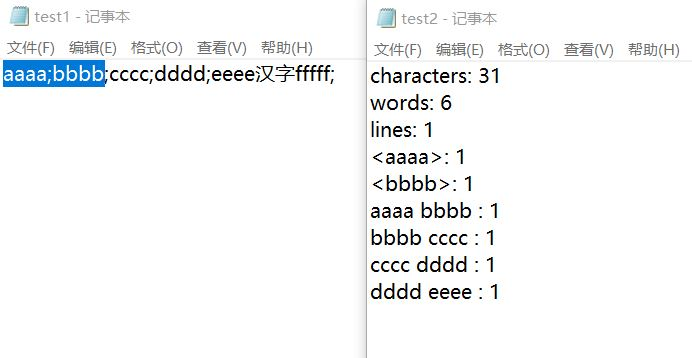posted @ 2019-10-13 21:48  im-iron_man  阅读(370)  评论(1编辑  收藏  举报